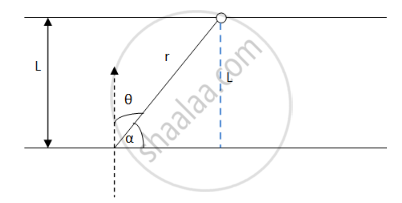Department of Pre-University Education, KarnatakaPUC Karnataka Science Class 12

# A Point Source of Light Moves in a Straight Line Parallel to a Plane Table. Consider a Small Portion of the Table Directly Below the Line of Movement of the Source. - Physics

MCQ
Fill in the Blanks

A point source of light moves in a straight line parallel to a plane table. Consider a small portion of the table directly below the line of movement of the source. The illuminance at this portion varies with its distance r from the source as ___________ .

#### Options

• $1 \propto \frac{1}{r}$

• $1 \propto \frac{1}{r^2}$

• $1 \propto \frac{1}{r^3}$

• $1 \propto \frac{1}{r^4}$

#### Solution

$1 \propto \frac{1}{r^3}$Let the distance between the parallel straight lines be L.

Angle with normal = ​θ

We know,

$I = \frac{I_o \cos\theta}{r^2}$

From the above figure, we  get

$I = \frac{I_o \cos\left( {90}^0 - \alpha \right)}{r^2}$

$\Rightarrow I = \frac{I_o \sin\alpha}{r^2}$

$\Rightarrow I = \frac{I_o}{r^2}\left( \frac{L}{r} \right)$

$\Rightarrow I = \frac{I_o L}{r^3}$

L =  constant for parallel moving source

So, $I_o L = k ............\left(\text{constant}\right)$

$\Rightarrow I = \frac{k}{r^3}$

$\Rightarrow I\alpha\frac{1}{r^3}$

Concept: Light Process and Photometry
Is there an error in this question or solution?

#### APPEARS IN

HC Verma Class 11, Class 12 Concepts of Physics Vol. 1
Chapter 22 Photometry
MCQ | Q 10 | Page 454
Share